# 基于深度学习YOLOv2算法的钢材压印字符识别研究Research on Character Recognition of Steel Embossing Based on YOLOv2

• 全文下载: PDF(1137KB)    PP.126-135   DOI: 10.12677/CSA.2020.101014
• 下载量: 63  浏览量: 99   国家自然科学基金支持

Aiming at the influence of the same color as the background area of industrial parts and uneven illumination of the steel embossing characters, there is a problem of poor efficiency and precision of traditional computer vision algorithms to identify steel embossing characters. This research proposes a steel embossing character recognition method based on YOLOv2. Through some basic image preprocessing methods, the steel embossing character data set is expanded, and the fast and reliable deep learning algorithm YOLOv2 is used to automatically extract the features of the image to realize the recognition of the steel embossing characters (including numbers and letters). Compared with other traditional image recognition algorithms, the experimental results show that the accuracy of the network model for the identification of steel embossing characters is 98.6%, and the average processing time of the algorithm is 0.3 s, which meets the accuracy and efficiency requirements of engineering applications. In addition, the output of the model is improved by using the character position information, and the correct production label can be output directly. It has good stability and real-time performance in industrial production environment and has certain application significance.

1. 引言

2. 识别方法

2.1. 图像预处理

2.1.1. 倾斜矫正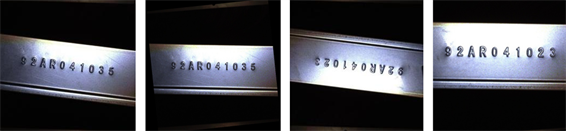(a) 原始图像 (b) 倾斜矫正 (c) 倒置原始图像 (d) 旋转矫正

Figure 1. Tilt correction picture

2.1.2. 图像增强

$y=med\left({x}_{1},{x}_{2},\cdots ,{x}_{n}\right)=\left\{\begin{array}{l}{x}_{\frac{n+1}{2}},\text{\hspace{0.17em}}\text{\hspace{0.17em}}n为奇数\\ \frac{1}{2}\left({x}_{\frac{n}{2}}+{x}_{\frac{n}{2}+1}\right),\text{\hspace{0.17em}}\text{\hspace{0.17em}}n为偶数\end{array}$ (1)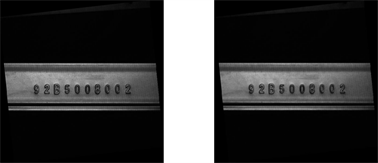(a) 灰度化 (b) 中值滤波

Figure 2. Image enhancement effect map

2.1.3. 数据集扩充

$\underset{f}{\mathrm{min}}{\iint }_{\Omega }{|\nabla f-V|}^{2},{f|}_{\partial \Omega }={{f}^{*}|}_{\partial \Omega }$ (2)

$\Delta f=divV,{f|}_{\partial \Omega }={{f}^{*}|}_{\partial \Omega }$ (3)

2.2. YOLOv2原理

2.2.1. YOLOv2网络结构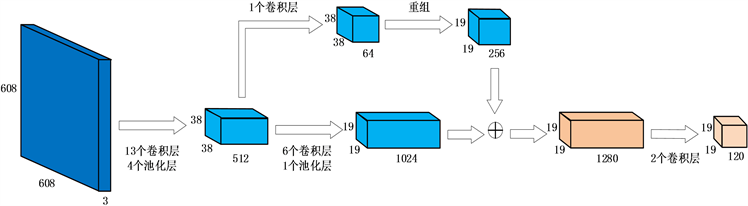Figure 3. The network structure of YOLOv2

2.2.2. 检测过程

YOLOv2在对钢材压印字符检测识别的过程中，会将整个输入图像划分成 $S×S$ 的网格，每个网格负责检测中心点落在网格中的对象。同时，YOLOv2网络借鉴Faster R-CNN的思想，引入Anchor Boxes先验框，不同于Fast R-CNN需要人工定义Anchor Boxes，YOLOv2采用K-means聚类方法对数据集中的人工标记框进行聚类分析，确定Anchor boxes的数量和大小，最后在网格的周围生成几个一定比例的边框。那么，每个网格单元预测B个边界框和这些框的置信度分数，每个边界框包含了该区域中心点的位置(x, y)，高度h，宽度w和置信度confidence这5个信息。

$confidence=P\left(object\right)×IO{U}_{pred}^{truth}$ (4)

$IO{U}_{pred}^{truth}=\frac{Area\left(pred\right)\cap Area\left(truth\right)}{Area\left(pred\right)\cup Area\left(truth\right)}$ (5)

$\begin{array}{c}Con{f}_{i}=P\left(clas{s}_{i}|object\right)×P\left(object\right)×IO{U}_{pred}^{truth}\\ =P\left(clas{s}_{i}\right)×IO{U}_{pred}^{truth}\end{array}$ (6)

2.2.3. 输出改进

3. 实验结果及分析

3.1. 实验平台

3.2. 训练

YOLOv2网络在训练时可以通过计算损失函数Loss来判断训练的效果，计算方法在等式(7)中示出。

$\begin{array}{c}Loss={\lambda }_{coord}\underset{i=0}{\overset{{S}^{2}}{\sum }}\underset{j=0}{\overset{B}{\sum }}{I}_{i,j}^{obj}\left[{\left({x}_{i}-{\stackrel{^}{x}}_{i}\right)}^{2}+{\left({y}_{i}-{\stackrel{^}{y}}_{i}\right)}^{2}\right]\text{\hspace{0.17em}}\text{\hspace{0.17em}}+{\lambda }_{coord}\underset{i=0}{\overset{{S}^{2}}{\sum }}\underset{j=0}{\overset{B}{\sum }}{I}_{i,j}^{obj}\left[{\left(\sqrt{{w}_{i}}-\sqrt{{\stackrel{^}{w}}_{i}}\right)}^{2}+{\left(\sqrt{{h}_{i}}-\sqrt{{\stackrel{^}{h}}_{i}}\right)}^{2}\right]\\ \text{\hspace{0.17em}}\text{\hspace{0.17em}}+\underset{i=0}{\overset{{S}^{2}}{\sum }}\underset{j=0}{\overset{B}{\sum }}{I}_{i,j}^{obj}{\left({C}_{i}-{\stackrel{^}{C}}_{i}\right)}^{2}+{\lambda }_{coobj}\underset{i=0}{\overset{{S}^{2}}{\sum }}\underset{j=0}{\overset{B}{\sum }}{I}_{i,j}^{noobj}{\left({C}_{i}-{\stackrel{^}{C}}_{i}\right)}^{2}\text{\hspace{0.17em}}\text{\hspace{0.17em}}+\underset{i=0}{\overset{{S}^{2}}{\sum }}{I}_{i}^{obj}\underset{c\in classes}{\overset{{S}^{2}}{\sum }}{\left({p}_{i}\left(c\right)-{\stackrel{^}{p}}_{i}\left(c\right)\right)}^{2}\end{array}$ (7)

YOLOv2网络在训练过程中还采用了多尺度训练策略，每隔十轮便改变一次图像尺寸进行输入，以提高模型对不同分辨率图像的鲁棒性。使用YOLO v2网络在钢材压印字符训练集训练中随着迭代次数的增加平均损失率所产生的变化如图4所示，从图中可以看出网络收敛速度较快，在迭代2000次左右时基本收敛，之后处于基本稳定状态，并且Loss值越来越接近于0，说明训练的效果逐渐趋于最佳。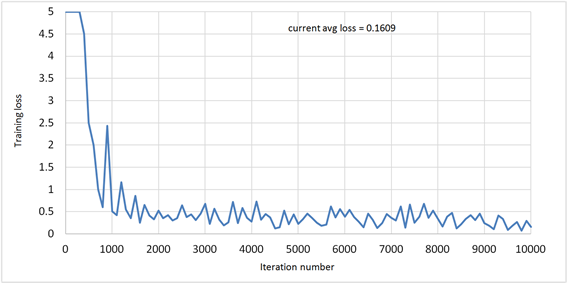Figure 4. Loss rate curve

3.3. 测试结果及分析Table 1. Performance indicators for recognition assessment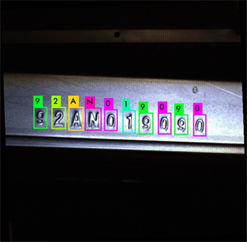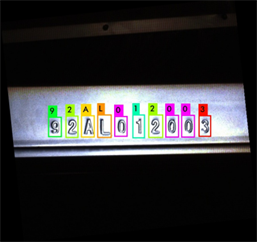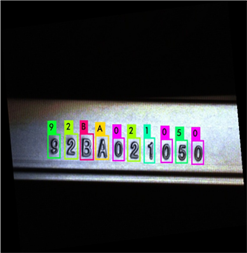Figure 5. Recognition effect map

4. 结论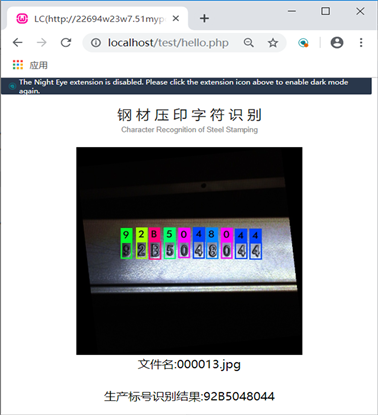Figure 6. Steel stamping character recognition platform based on YOLOv2

  顾晨勤, 葛万成. 基于模板匹配算法的字符识别研究[J]. 通信技术, 2009, 42(3): 220-222.  Lin, T.-Y., Dollar, P., Girshick, R., He, K., Hariharan, B. and Belongie, S. (2017) Feature Pyramid Networks for Object Detection. Pro-ceedings of the IEEE Conference on Computer Vision and Pattern Recognition, Honolulu, 21-26 July 2017, 936-944. https://doi.org/10.1109/CVPR.2017.106  Felzenszwalb, P.F., Girshick, R.B., Mcallester, D., et al. (2009) Ob-ject Detection with Discriminatively Trained Part-Based Models. IEEE Transactions on Pattern Analysis and Machine Intelligence, 32, 1627-1645. https://doi.org/10.1109/TPAMI.2009.167  Dalal, N. and Triggs, B. (2005). Histograms of Oriented Gradients for Human Detection. Proceedings of the IEEE Computer Society Conference on Computer Vision and Pattern Recogni-tion, San Diego, 20-25 June 2005, 886-893. https://doi.org/10.1109/CVPR.2005.177  Cortes, C. and Vapnik, V. (1995) Support-Vector Networks. Ma-chine Learning, 20, 273-297. https://doi.org/10.1007/BF00994018  Zhu, Q., Yeh, M.-C., Cheng, K.-T. and Avidan, S. (2006) Fast Human Detection Using a Cascade of Histograms of Oriented Gradients. Proceedings of the IEEE Computer Society Conference on Computer Vision and Pattern Recognition, New York, 17-22 June 2006, 1491-1498. https://doi.org/10.1109/CVPR.2006.119  Wang, X., Yang, M., Zhu, S. and Lin, Y. (2013) Regionlets for Ge-neric Object Detection. Proceedings of the IEEE International Conference on Computer Vision, Sydney, 1-8 December 2013, 17-24. https://doi.org/10.1109/ICCV.2013.10  Azizpour, H. and Laptev, I. (2012) Object Detection Using Strong-ly-Supervised Deformable Part Models. Proceedings of the European Conference on Computer Vision, Florence, 7-13 October 2012, 836-849. https://doi.org/10.1007/978-3-642-33718-5_60  Girshick, R., Donahue, J., Darrell, T. and Malik, J. (2014) Rich Feature Hierarchies for Accurate Object Detection and Semantic Segmentation. Proceedings of the IEEE Conference on Computer Vision and Pattern Recognition, Columbus, 23-28 June 2014, 580-587. https://doi.org/10.1109/CVPR.2014.81  Uijlings, J.R., Van De Sande, K.E., Gevers, T., et al. (2013) Selective Search for Object Recognition. International Journal of Computer Vision, 104, 154-171. https://doi.org/10.1007/s11263-013-0620-5  Girshick, R. (2015) Fast R-CNN. Proceedings of the IEEE Inter-national Conference on Computer Vision, Santiago, 7-13 December 2015, 1440-1448. https://doi.org/10.1109/ICCV.2015.169  Ren, S.Q., He, K.M., Girshick, R., et al. (2015) Faster R-CNN: To-wards Real-Time Object Detection with Region Proposal Networks. Advances in Neural Information Processing Systems, 28, 91-99.  Redmon, J., Divvala, S., Girshick, R. and Farhadi, A. (2016) You Only Look Once: Unified, Real-Time Object Detection. Proceedings of the IEEE Conference on Computer Vision and Pattern Recognition, Las Vegas, 27-30 June 2016, 779-788. https://doi.org/10.1109/CVPR.2016.91  Redmon, J. and Farhadi, A. (2017) YOLO9000: Better, Faster, Stronger. Proceedings of the IEEE Conference on Computer Vision and Pattern Recognition, Honolulu, 21-26 July 2017, 6517-6525. https://doi.org/10.1109/CVPR.2017.690  Nodes, T. and Gallagher, N.C.J. (1982) Median Filters: Some Modi-fications and Their Properties. IEEE Transactions on Acoustics, Speech, and Signal Processing, 30, 739-746. https://doi.org/10.1109/TASSP.1982.1163951  Pérez, P., Gangnet, M. and Blake, A. (2003) Poisson Image Editing. ACM Transactions on Graphics, 22, 313-318. https://doi.org/10.1145/882262.882269  Simonyan, K. and Zisserman, A. (2015) Very Deep Convolutional Networks for Large-Scale Image Recognition. International Conference on Learning Representations, San Diego, 7-9 May 2015, 1-14.  Szegedy, C., Liu, W., Jia, Y., et al. (2015) Going Deeper with Convolutions. Proceedings of the IEEE Conference on Computer Vision and Pattern Recognition, Boston, 7-12 June 2015, 1-9. https://doi.org/10.1109/CVPR.2015.7298594  Lin, M., Chen, Q. and Yan, S.C. (2014) Network in Net-work.  Lecun, Y., Bottou, L., Bengio, Y., et al. (1998) Gradient-Based Learning Applied to Document Recognition. Proceedings of the IEEE, 86, 2278-2324. https://doi.org/10.1109/5.726791